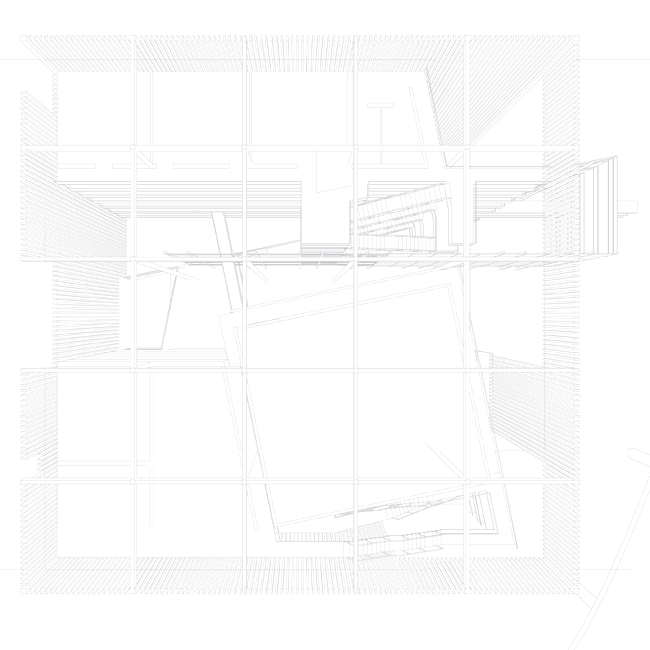P o i n t,   L i n e,   P l a n e :  Basic Elements of Formal Composition in Bauhaus and Shape Computation TheoriesKotsopoulos S, S.M. Thesis, Massachusetts Institute of Technology, 2000
 C o n s t r u c t i n g   D e s i g n   C o n c e p t s :   A Computational Approach to the Synthesis of Architectural Form Kotsopoulos S, Ph.D. Dissertation, Massachusetts Institute of Technology, 2005
 S  o  t  i  r  i  o  s     D.     K  o  t  s  o  p  o  u   l  o  s           D i p l.     A r c h - E n g        M A r c h       S M A r c h       P h D
 r e s e a r c h